# Community

Blog Events Webinars Tutorials Forum
×
Community Blog Image Classification with TensorFlow

# Image Classification with TensorFlow

This article introduces a method of image recognition using deep learning that can be applied to image filtering, and object detection.

11.11 The Biggest Deals of the Year. 40% OFF on selected cloud servers with a free 100 GB data transfer! Click here to learn more.

Effectively making use of unstructured data from large amounts of image and voice data has always been a challenge for data mining professionals. The processing of unstructured data usually involves the use of deep learning algorithms and these algorithms can be daunting for beginners. In addition, processing unstructured data usually requires powerful GPUs and a large amount of computing resources. This article introduces a method of image recognition using deep learning. This method can be applied to scenarios such as illicit image filtering, and object detection.

This experiment creates an image recognition model using the deep learning framework TensorFlow in Alibaba Cloud Machine Learning Platform for AI. The entire procedure takes about 30 minutes to complete. After the model training process, the system is able to recognize the bird in the following image, and return the word "bird":This experiment can be created from the following TensorFlow image classification template: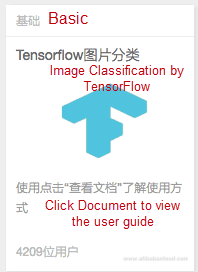If you choose to create the experiment from the template, replace the checkpoint path in both the parent and child TensorFlow components with your OSS paths and then run the experiment, as shown in the following figure: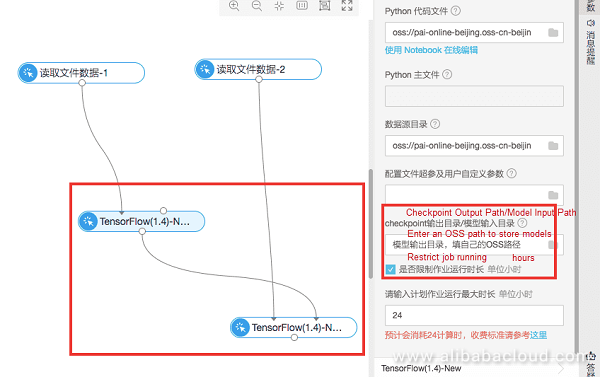## Datasets

You can download the dataset and corresponding code used in this experiment from https://help.aliyun.com/document_detail/51800.html.

A CIFAR-10 dataset is used in this experiment. This dataset contains 60,000 images with pixel dimensions of 32 x 32. These images are classified into 10 categories, including airplanes, cars, birds, cats, deer, dogs, frogs, horses, ships, and trucks. The following figure shows the dataset: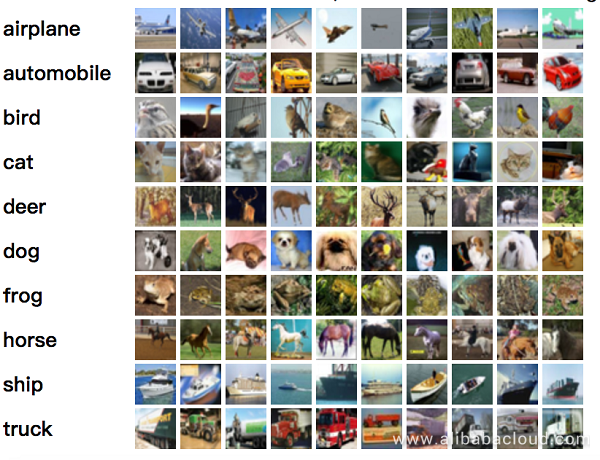The dataset is divided into two parts: 50,000 images are used for training and 10,000 for testing. The 50,000 training images are divided into five data_batch files, and the 10,000 testing images are added to file test_batch. The training and testing data files are as follows: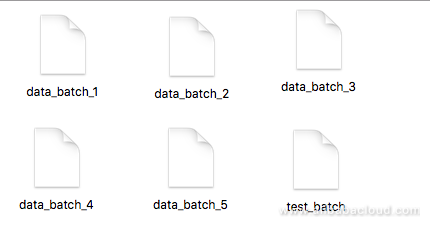## Data Exploration

To create the experiment in the Alibaba Cloud Machine Learning Platform for AI, you need to enable GPU usage and activate Object Storage Service (OSS) to store your data.

### 1. Data Source Settings

First, log in to OSS, and upload the dataset and source code to an OSS bucket. To perform this task, create an OSS bucket, create folder aohai_test in the bucket, and then create four folders in the aohai_test folder, as follows: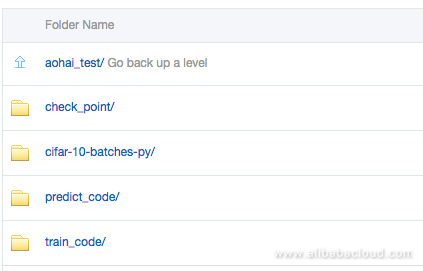The usage of the folders is as follows:

1. check_point: stores the model that is generated in the experiment.
2. cifar-10-batches-py: stores training data file cifar-10-batcher-py and prediction data file bird_mount_bluebird.jpg.
3. train_code: stores code file cifar_pai.py.
4. predict_code: stores code file cifar_predict_pai.py.

### 2. OSS Access Authorization

After uploading the data and code to OSS, you need to grant Alibaba Cloud Machine Learning Platform for AI access permissions to OSS. Log in to the Alibaba Cloud Machine Learning Platform for AI console, click Settings in the left-side navigation pane, and then grant access permissions, as shown in the following figure: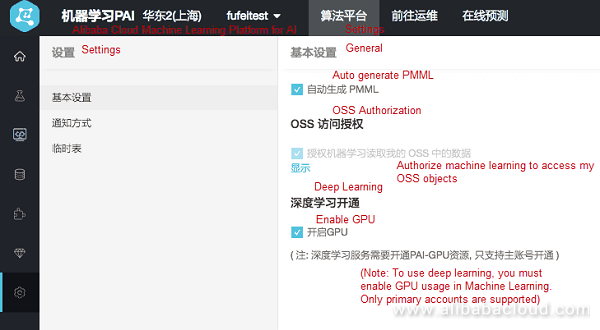### 3. Model Training Logic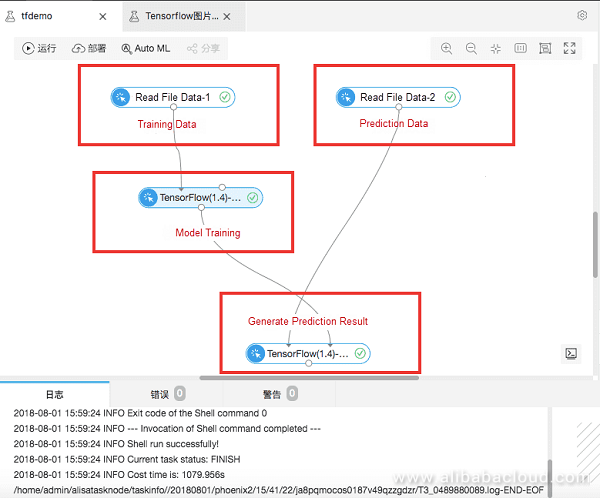### 4. Training and Prediction Data Settings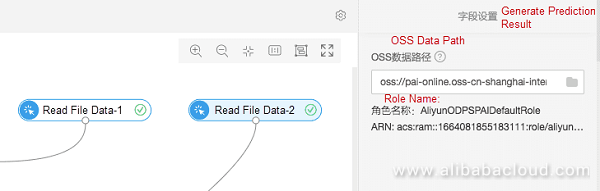Set the OSS paths that store the training data and prediction data.

### 5. Model Training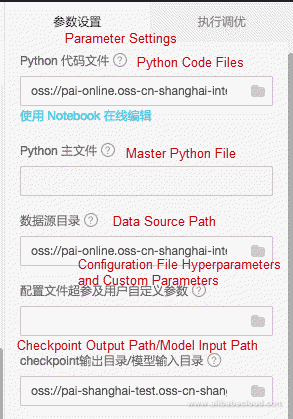1. Python Code File: select file cifar_pai.py stored on OSS.
2. Data Source Path: select folder cifar-10-batches-py on OSS. The data is automatically synchronized from the parent read file data component.
3. Checkpoint Output Path/Model Input Path: select folder check_point in OSS to store the models.
4. Tuning: specify the deployment mode (centralized or distributed) and the number of GPUs.

Code parsing

The key code in file cifar_pai.py is as follows:

1. The following code generates a Convolutional Neural Network (CNN) image classification model.
``````1.    network = input_data(shape=[None, 32, 32, 3],
2.                             data_preprocessing=img_prep,
3.                             data_augmentation=img_aug)
4.        network = conv_2d(network, 32, 3, activation='relu')
5.        network = max_pool_2d(network, 2)
6.        network = conv_2d(network, 64, 3, activation='relu')
7.        network = conv_2d(network, 64, 3, activation='relu')
8.        network = max_pool_2d(network, 2)
9.        network = fully_connected(network, 512, activation='relu')
10.        network = dropout(network, 0.5)
11.        network = fully_connected(network, 10, activation='softmax')
13.                             loss='categorical_crossentropy',
14.                             learning_rate=0.001)``````

2. The following code generates model model.tfl.
``````1.        model = tflearn.DNN(network, tensorboard_verbose=0)
2.        model.fit(X, Y, n_epoch=100, shuffle=True, validation_set=(X_test, Y_test),
3.                  show_metric=True, batch_size=96, run_id='cifar10_cnn')
4.        model_path = os.path.join(FLAGS.checkpointDir, "model.tfl")
5.        print(model_path)
6.        model.save(model_path)``````

Code parsing

You can right-click the TensorFlow component to view the log that is generated during the training process.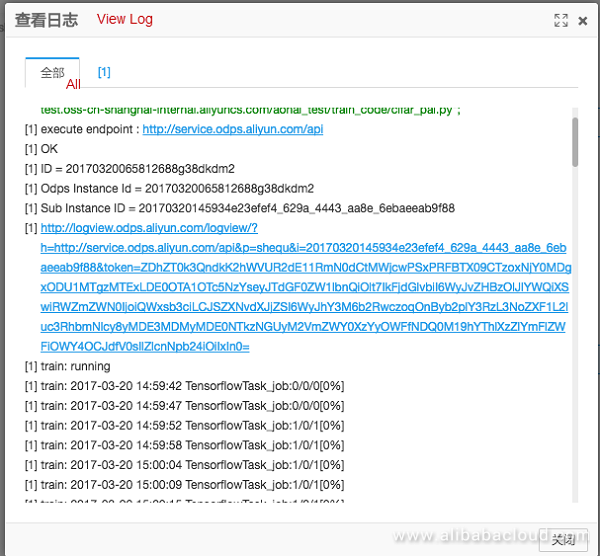3. Click Terminated, and then click StdOut to output the model training log in real time.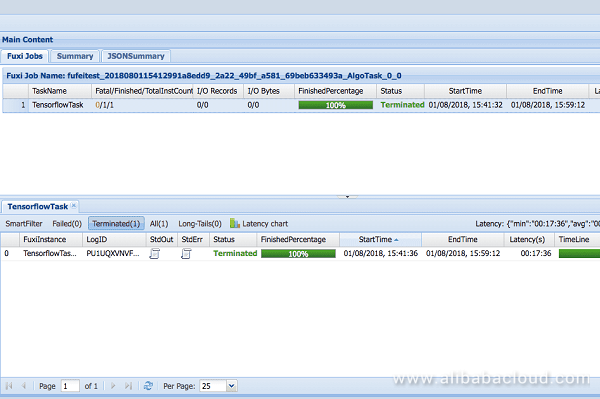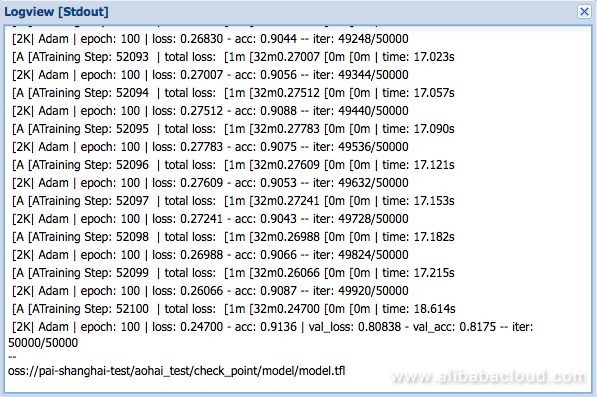More information is output as the experiment runs. You can also use the print function in the code to output the key information in logview. In this experiment, you can use the aac parameter to view the accuracy of model training.

## Making Predictions

You can drag and drop another TensorFlow component for making predictions.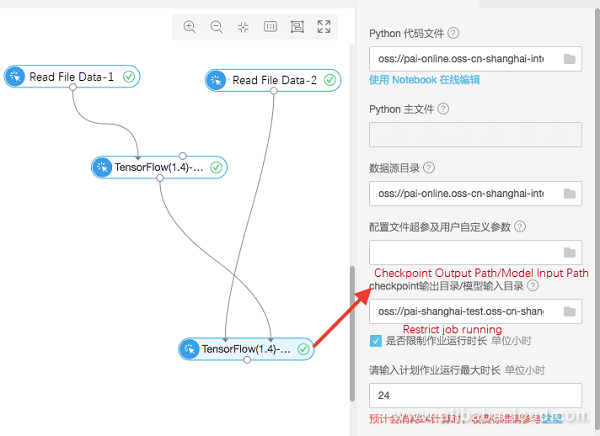1. Python Code File: select file cifar_predict_pai.py stored on OSS.
2. Data Source Path: select folder cifar-10-batches-py on OSS to read file bird_mount_bluebird.jpg. The data is automatically synchronized from the parent read file component.
3. Checkpoint Output Path/Model Input Path: select the same folder that is used to store the models.

The image that is used for prediction is stored in the checkpoint folder:The prediction result is contained in the corresponding log. To view the log, see step 4.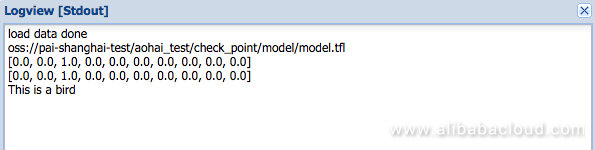Prediction code

The following shows a portion of the prediction code:

``````1.      predict_pic = os.path.join(FLAGS.buckets, "bird_bullocks_oriole.jpg")
3.        file_io.write_string_to_file("bird_bullocks_oriole.jpg", img_obj)
4.
6.
7.        # Scale it to 32x32
8.        img = scipy.misc.imresize(img, (32, 32), interp="bicubic").astype(np.float32, casting='unsafe')
9.
10.        # Predict
11.        prediction = model.predict([img])
12.        print (prediction)
13.        print (prediction)
14.        #print (prediction.index(max(prediction)))
15.        num=['airplane','automobile','bird','cat','deer','dog','frog','horse','ship','truck']
16.        print ("This is a %s"%(num[prediction.index(max(prediction))]))``````

1. Reads image bird_bullocks_oriole.jpg, and scales the image to 32 x 32 pixels.
2. Passes the image to the 'model.predict' function to calculate the weights of the image in the following ten categories: 'airplane', 'automobile', 'bird', 'cat', 'deer', 'dog', 'frog', 'horse', 'ship', and 'truck'.
3. Returns the category with the largest weight as the prediction result.

0 0 0
Share on

# Related Products

• ##AIRec

A high-quality personalized recommendation service for your applications.

• ##Artificial Intelligence Service for Conversational Chatbots Solution

This solution provides you with Artificial Intelligence services and allows you to build AI-powered, human-like, conversational, multilingual chatbots over omnichannel to quickly respond to your customers 24/7.

• ##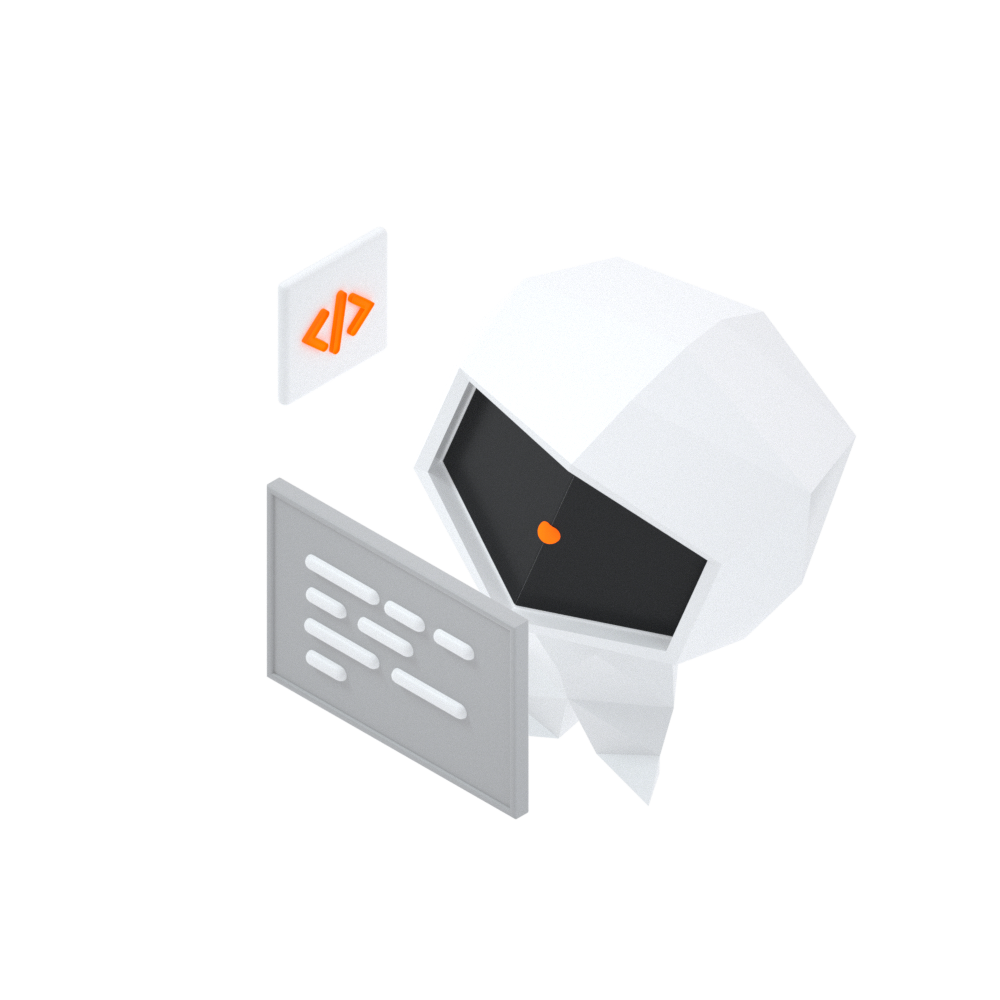Log Management for AIOps Solution

Log into an artificial intelligence for IT operations (AIOps) environment with an intelligent, all-in-one, and out-of-the-box log management solution

• ##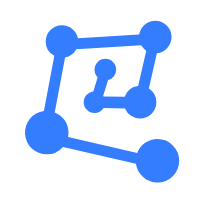Machine Learning Platform For AI

An end-to-end platform that provides various machine learning algorithms to meet your data mining and analysis requirements.